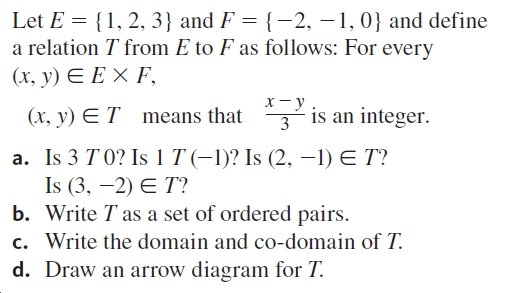# Let E = {1, 2, 3} and F = {-2, –1, 0} and definea relation T from E to F as follows: For every(х, у) € ЕХ F,х —у3 is an integer.(x, y) ETmeans thata. Is 3 T 0? Is 1 T (-1)? Is (2, -1) E T?Is (3, –2) E T?b. Write T as a set of ordered pairs.c. Write the domain and co-domain of T.d. Draw an arrow diagram for T.

Question
40 viewshelp_outlineImage TranscriptioncloseLet E = {1, 2, 3} and F = {-2, –1, 0} and define a relation T from E to F as follows: For every (х, у) € ЕХ F, х —у 3 is an integer. (x, y) ET means that a. Is 3 T 0? Is 1 T (-1)? Is (2, -1) E T? Is (3, –2) E T? b. Write T as a set of ordered pairs. c. Write the domain and co-domain of T. d. Draw an arrow diagram for T. fullscreen
check_circle

Step 1
Step 2
Step 3

### Want to see the full answer?

See Solution

#### Want to see this answer and more?

Solutions are written by subject experts who are available 24/7. Questions are typically answered within 1 hour.*

See Solution
*Response times may vary by subject and question.
Tagged in

### Math